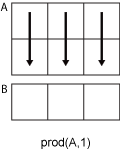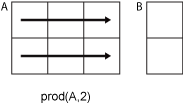# prod

Product of array elements

## Syntax

``B = prod(A)``
``B = prod(A,'all')``
``B = prod(A,dim)``
``B = prod(A,vecdim)``
``B = prod(___,outtype)``
``B = prod(___,nanflag)``

## Description

example

````B = prod(A)` returns the product of the array elements of `A`. If `A` is a vector, then `prod(A)` returns the product of the elements.If `A` is a nonempty matrix, then `prod(A)` treats the columns of `A` as vectors and returns a row vector of the products of each column. If `A` is an empty 0-by-0 matrix, `prod(A)` returns `1`.If `A` is a multidimensional array, then `prod(A)` acts along the first nonsingleton dimension and returns an array of products. The size of this dimension reduces to `1` while the sizes of all other dimensions remain the same. `prod` computes and returns `B` as `single` when the input, `A`, is `single`. For all other numeric and logical data types, `prod` computes and returns `B` as `double`.```

example

````B = prod(A,'all')` computes the product of all elements of `A`. This syntax is valid for MATLAB® versions R2018b and later.```

example

````B = prod(A,dim)` returns the products along dimension `dim`. For example, if `A` is a matrix, `prod(A,2)` is a column vector containing the products of each row.```

example

````B = prod(A,vecdim)` computes the product based on the dimensions specified in the vector `vecdim`. For example, if `A` is a matrix, then `prod(A,[1 2])` is the product of all elements in `A`, since every element of a matrix is contained in the array slice defined by dimensions 1 and 2.```

example

````B = prod(___,outtype)` returns an array in the class specified by `outtype`, using any of the input arguments in the previous syntaxes. `outtype` can be `'double'`, `'native'`, or `'default'`.```

example

````B = prod(___,nanflag)` specifies whether to include or omit `NaN` values from the calculation for any of the previous syntaxes. `prod(A,'includenan')` includes `NaN` values in the calculation while `prod(A,'omitnan')` ignores them.```

## Examples

collapse all

Create a 3-by-3 array whose elements correspond to their linear indices.

`A=[1:3:7;2:3:8;3:3:9]`
```A = 3×3 1 4 7 2 5 8 3 6 9 ```

Find the product of the elements in each column. The length of the first dimension is 1, and the length of the second dimension matches `size(A,2)`.

`B = prod(A)`
```B = 1×3 6 120 504 ```

Create an array of logical values.

`A = [true false; true true]`
```A = 2x2 logical array 1 0 1 1 ```

Find the product of the elements in each column.

`B = prod(A)`
```B = 1×2 1 0 ```

The output has type `double`.

`class(B)`
```ans = 'double' ```

Create a 3-by-3 array whose elements correspond to their linear indices.

`A=[1:3:7;2:3:8;3:3:9]`
```A = 3×3 1 4 7 2 5 8 3 6 9 ```

Find the product of the elements in each row and reduce the length of the second dimension to 1. The length of the first dimension matches `size(A,1)`, and the length of the second dimension is 1.

```dim = 2; B = prod(A,dim)```
```B = 3×1 28 80 162 ```

Create a 3-D array and compute the product over each page of data (rows and columns).

```A(:,:,1) = [2 4; -2 1]; A(:,:,2) = [1 2; -5 3]; A(:,:,3) = [4 4; 1 -3]; B1 = prod(A,[1 2])```
```B1 = B1(:,:,1) = -16 B1(:,:,2) = -30 B1(:,:,3) = -48 ```

To compute the product over all dimensions of an array, you can either specify each dimension in the vector dimension argument, or use the `'all'` option.

`B2 = prod(A,[1 2 3])`
```B2 = -23040 ```
`Ball = prod(A,'all')`
```Ball = -23040 ```

Create a 3-by-3 array of single-precision values.

`A = single([1200 1500 1800; 1300 1600 1900; 1400 1700 2000])`
```A = 3x3 single matrix 1200 1500 1800 1300 1600 1900 1400 1700 2000 ```

Find the product of the elements in each row by multiplying in double precision.

`B = prod(A,2,'double')`
```B = 3×1 109 × 3.2400 3.9520 4.7600 ```

The output is double precision.

`class(B)`
```ans = 'double' ```

Create a 3-by-3 array of 8-bit unsigned integers.

`A = uint8([1:3:7;2:3:8;3:3:9])`
```A = 3x3 uint8 matrix 1 4 7 2 5 8 3 6 9 ```

Find the product of the elements in each column natively in `uint8`.

`B = prod(A,'native')`
```B = 1x3 uint8 row vector 6 120 255 ```

The result is an array of 8-bit unsigned integers.

`class(B)`
```ans = 'uint8' ```

Create a vector and compute its product, excluding `NaN` values. If you do not specify `'omitnan'`, then `prod(A)` returns `NaN`.

```A = [1 3 2 4 NaN 3 NaN 2]; P = prod(A,'omitnan')```
```P = 144 ```

## Input Arguments

collapse all

Input array, specified as a vector, matrix, or multidimensional array.

Data Types: `double` | `single` | `int8` | `int16` | `int32` | `int64` | `uint8` | `uint16` | `uint32` | `uint64` | `logical`
Complex Number Support: Yes

Dimension to operate along, specified as a positive integer scalar. If you do not specify the dimension, then the default is the first array dimension of size greater than 1.

Dimension `dim` indicates the dimension whose length reduces to `1`. The `size(B,dim)` is `1`, while the sizes of all other dimensions remain the same.

Consider a two-dimensional input array, `A`.

• If `dim = 1`, then `prod(A,1)` returns a row vector containing the product of the elements in each column.• If `dim = 2`, then `prod(A,2)` returns a column vector containing the product of the elements in each row.`prod` returns `A` when `dim` is greater than `ndims(A)`.

Data Types: `double` | `single` | `int8` | `int16` | `int32` | `int64` | `uint8` | `uint16` | `uint32` | `uint64`

Vector of dimensions, specified as a vector of positive integers. Each element represents a dimension of the input array. The lengths of the output in the specified operating dimensions are 1, while the others remain the same.

Consider a 2-by-3-by-3 input array, `A`. Then `prod(A,[1 2])` returns a 1-by-1-by-3 array whose elements are the products of each page of `A`.Data Types: `double` | `single` | `int8` | `int16` | `int32` | `int64` | `uint8` | `uint16` | `uint32` | `uint64`

Output class, specified as `'default'`, `'double'`, or `'native'`, and which defines the data type of the output, `B`.

`outtype`Output data type
`'default'``double`, unless the input data type is `single`. In which case, the output data type is `single`.
`'double'``double`
`'native'`same data type as the input array, `A`

`NaN` condition, specified as one of these values:

• `'includenan'` — Include `NaN` values from the input when computing the product, resulting in `NaN` output.

• `'omitnan'` — Ignore `NaN` values in the input. If all elements are `NaN`, then `prod` returns 1.

## Output Arguments

collapse all

Product array, returned as a scalar, vector, matrix, or multidimensional array.

The class of `B` is as follows:

• If the `outtype` argument specifies `'default'` or is not used

• and the input is not `single`, then the output is `double`.

• and the input is `single`, then the output is `single`.

• If the `outtype` argument specifies `'double'`, then the output is `double` regardless of the input data type.

• If the `outtype` argument specifies `'native'`, then the output is the same data type as the input.

collapse all

### First Nonsingleton Dimension

The first nonsingleton dimension is the first dimension of an array whose size is not equal to `1`.

For example:

• If `X` is a 1-by-n row vector, then the second dimension is the first nonsingleton dimension of `X`.

• If `X` is a 1-by-0-by-n empty array, then the second dimension is the first nonsingleton dimension of `X`.

• If `X` is a 1-by-1-by-3 array, then the third dimension is the first nonsingleton dimension of `X`.

## Version History

Introduced before R2006a